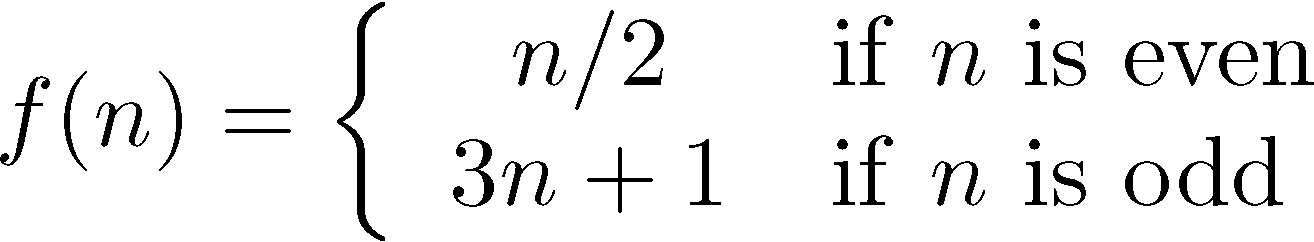# Homework #4

Due: May 30, 2014
Points: 100

This homework has you implement a function iteratively, then recursively, and then time them.

The Collatz sequence is the sequence of numbers generated by repeatedly computing the value of the functionfor n a positive integer, and stopping when n = 1.

So, for example, the Collatz sequence that begins with n = 6 is:

```
6, 3, 10, 5, 16, 8, 4, 2, 1
```
and the one that begins with n = 11 is:
```
11, 34, 17, 52, 26, 13, 40, 20, 10, 5, 16, 8, 4, 2, 1
```

1. (25 points) Write an iterative function itercol(n) that takes one argument, n, and prints the Collatz sequence that begins with n.

Input. This is a function, so it must take a positive integer as its only parameter. You do not need to write a wrapping program to call it, nor do you need to check that the parameter is a positive integer; you may assume that.

Output. Print the Collatz sequence beginning with n. For example, the function call

```
itercol(6)
```
is to produce
```
6, 3, 10, 5, 16, 8, 4, 2, 1
```

Submit. Name your file “itercol.py” and submit it to the Homework #4 area for this class on SmartSite.

2. (45 points) Now write a recursive function reccol(n) that takes one argument, n, and prints the Collatz sequence that begins with n. Remember, this function must call itself! Also, you do not need any loops – if you have one, you probably did it wrong.

Input. This is a function, so it must take a positive integer as its only parameter. You do not need to write a wrapping program to call it, nor do you need to check that the parameter is a positive integer; you may assume that.

Output. Print the Collatz sequence beginning with n. For example, the function call

```
reccol(6)
```
is to produce
```
6, 3, 10, 5, 16, 8, 4, 2, 1
```

Submit. Name your file “reccol.py” and submit it to the Homework #4 area for this class on SmartSite.

3. (30 points) Now you are to time the two functions and print out the timings. We will have to change your functions slightly to make the output manageable.

First, put both functions into a single file, and comment out the print statements in both functions. If you don’t comment them out, you will get lots of output that you do not want.

Then, after the functions, read in the initial number for generating the sequences. Here, check that it is a positive integer. If it is not, give an error message and exit the program. (See below.)

Use the time.clock() function to time each function. To get a good value, call each function 10,000 times, noting the time before the calls and the time after the calls. Then divide the time by 10,000 to get the average time per call to the function. Print the average time the iterative function takes, the average time the recursive function takes, andwhich is faster and by how much (see below).

Input. Your program asks the user for a number, reads it in and checks that it is a positive integer, exiting with an error message if not.

Here is what a correct input should look like (the red text is what you type):

```
Compute the Collatz sequence for this number: 27
```
If the input is invalid:
```
Compute the Collatz sequence for this number: hello
Need a non-negative integer
```
and then the program exits.

Output. The program is to print the time in seconds that the iterative version takes, the time in seconds that the recursive version takes, and the difference between the times.

Your output for the input 27 should look exactly like this:

```
Average iterative Collatz time: 2.29415e-05 seconds
Average recursive Collatz time: 3.31636e-05 seconds
Iterative version is faster by 1.02221e-05 seconds
```
except that your times, which is faster, and the difference, may vary.

When printing the times, and the difference of the times, use the %g format (not the %e or %f formats). When printing which is faster, say “Iterative version is faster by …”, “Recursive version is faster by …”, or “Recursive and iterative versions are equally fast”.

Submit. Name your file “coltimes.py” and submit it to the Homework #4 area for this class on SmartSite.

Hint: See section 12.2 of the textbook for examples on how to use time.clock() to time parts of your program.

 You can also obtain a PDF version of this. Version of May 21, 2014 at 9:12AM